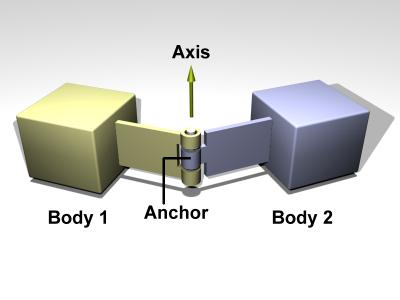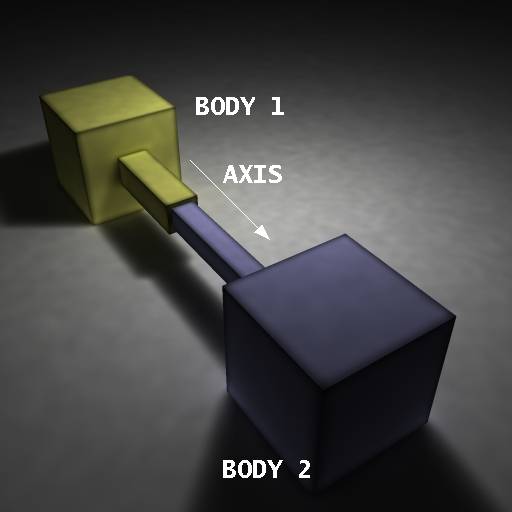# Joints¶

## Basics¶

Pyrosim makes use of 2 different types of joints:

1. Hinge Joints
2. Slider Joints

Hinge joints rotate around a joint axis at an anchor whereas slider joints slide along the direction of a joint axis.## Double Pendulum¶

This example creates a weighted double pendulum with a slider joint connecting the mass at the end. This example makes use of the body ID tags and introduces orientation in the cylinders (r1,r2,r3). Setting the debug tag to true when creating the simulator draws the hinge joints as red cylinders along the joint normals. Currently slider joints are not drawn.

dp-basic.py
import pyrosim
import math

sim = pyrosim.Simulator(play_paused=True, debug=True, use_textures=True,
xyz=[3.0, 2.0, 2.0], hpr=[-150.0, -25.0, 0.0],
eval_time=500)

# r1=1,r2=0, r3=0 means it is starting pointed along the x-axis
cyl_1 = sim.send_cylinder(x=-0.25, y=0.0, z=2.0,
r1=1, r2=0, r3=0,
cyl_2 = sim.send_cylinder(x=-0.75, y=0.0, z=2.0,
r1=1, r2=0, r3=0,

box = sim.send_box(x=-1.0, y=0.0, z=2.0,
width=0.5, length=0.5, height=0.5,
mass=10.0)

# hinge connects body to the world
# (x,y,z) refers to the anchor position
# (n1,n2,n3) refers to the joint axis
world_joint = sim.send_hinge_joint(first_body_id=pyrosim.Simulator.WORLD,
second_body_id=cyl_1,
x=0.0, y=0.0, z=2.0,
n1=0, n2=1, n3=0,
lo=-math.pi, hi=math.pi)

# hinge connects cylinders together
hinge_joint = sim.send_hinge_joint(first_body_id=cyl_1,
second_body_id=cyl_2,
x=-0.5, y=0.0, z=2.0,
n1=0, n2=1, n3=0,
lo=-math.pi, hi=math.pi)

# slider joint joins second cylinder and box.
# Contrary to the hinge joint, the parameters
# (x,y,z) refer to the joint axis in the case
# of the slider joint.
slider_joint = sim.send_slider_joint(first_body_id=cyl_2,
second_body_id=box,
x=1.0, y=0.0, z=0.0,
lo=-0.5, hi=0.5)

sim.start()
sim.wait_to_finish()